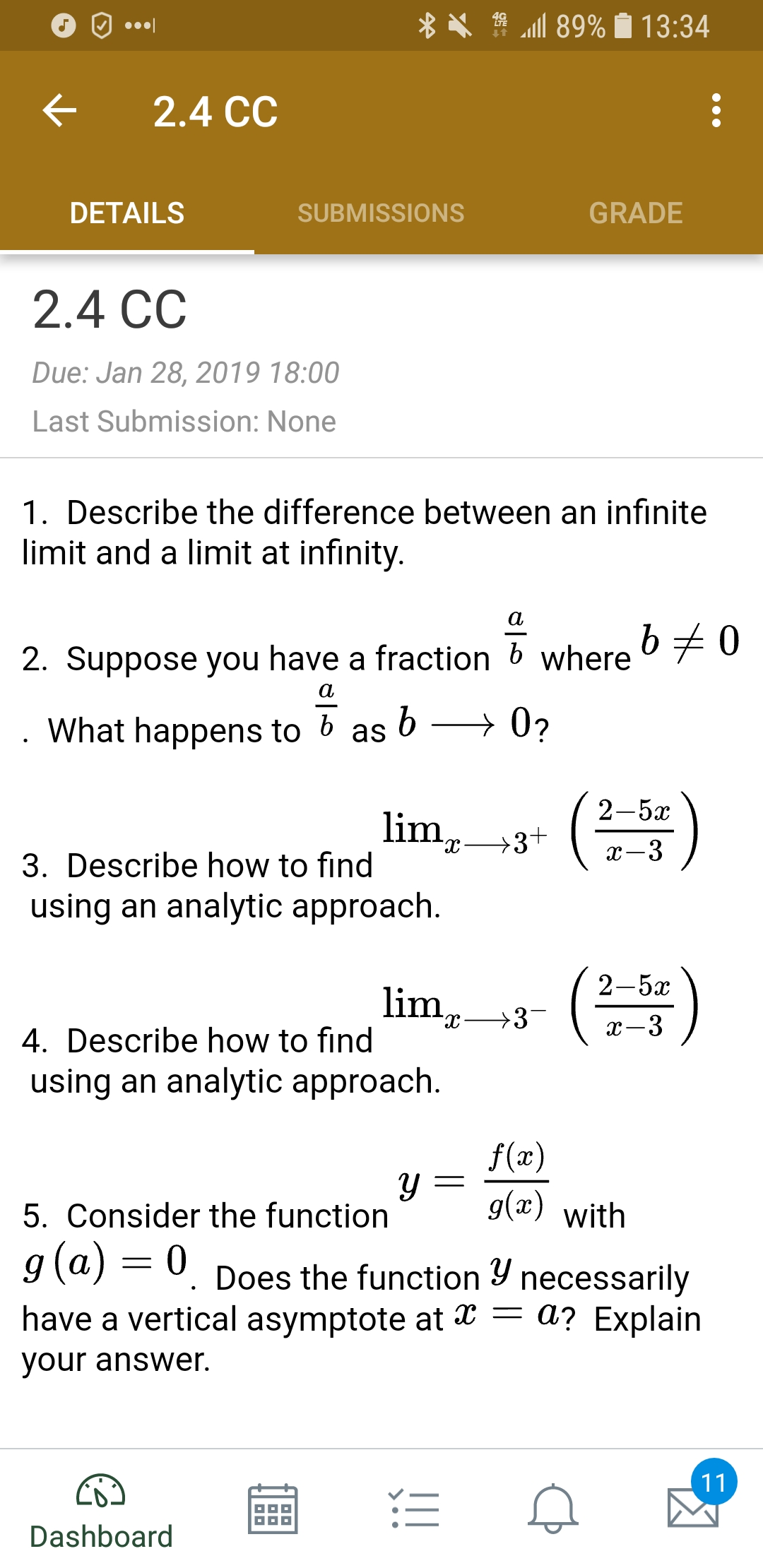# O. X . "all 89% 13:34 < 2.4 CC DETAILS SUBMISSIONS GRADE 2.4 CC Due: Jan 28, 2019 18:00 Last Submission: None 1. Describe the difference between an infinite limit and a limit at infinity 2. Suppose you have a fraction b where What happens to b as 2-5x 3. Describe how to find using an analytic approach 2-5x lim, ->3 dc 4. Describe how to find using an analytic approach f(x) y g(x) with 5. Consider the function 9(aj-0. Does the function y necessarily have a vertical asymptote at x - a? Explain our answer. Dashboard

Question

How would you determine the answer for number 5?help_outlineImage TranscriptioncloseO. X . "all 89% 13:34 < 2.4 CC DETAILS SUBMISSIONS GRADE 2.4 CC Due: Jan 28, 2019 18:00 Last Submission: None 1. Describe the difference between an infinite limit and a limit at infinity 2. Suppose you have a fraction b where What happens to b as 2-5x 3. Describe how to find using an analytic approach 2-5x lim, ->3 dc 4. Describe how to find using an analytic approach f(x) y g(x) with 5. Consider the function 9(aj-0. Does the function y necessarily have a vertical asymptote at x - a? Explain our answer. Dashboard fullscreen

### Want to see the step-by-step answer?